# 简介

• 基于规则特征匹配的方法（如根据喜欢，讨厌等特殊词来评判情感，但准确率低，通常作为一种辅助判断的方法）
• 基于传统机器学习的方法（特征工程 + 分类算法）
• 给予深度学习的方法（词向量 + 神经网络）

# TextRNN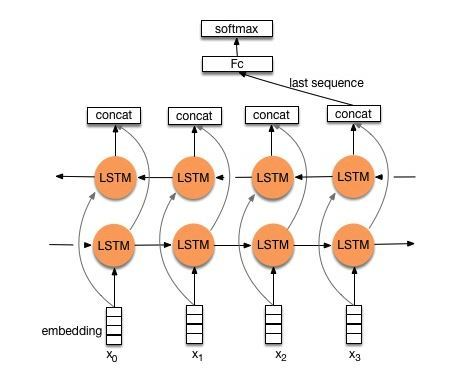# TextCNN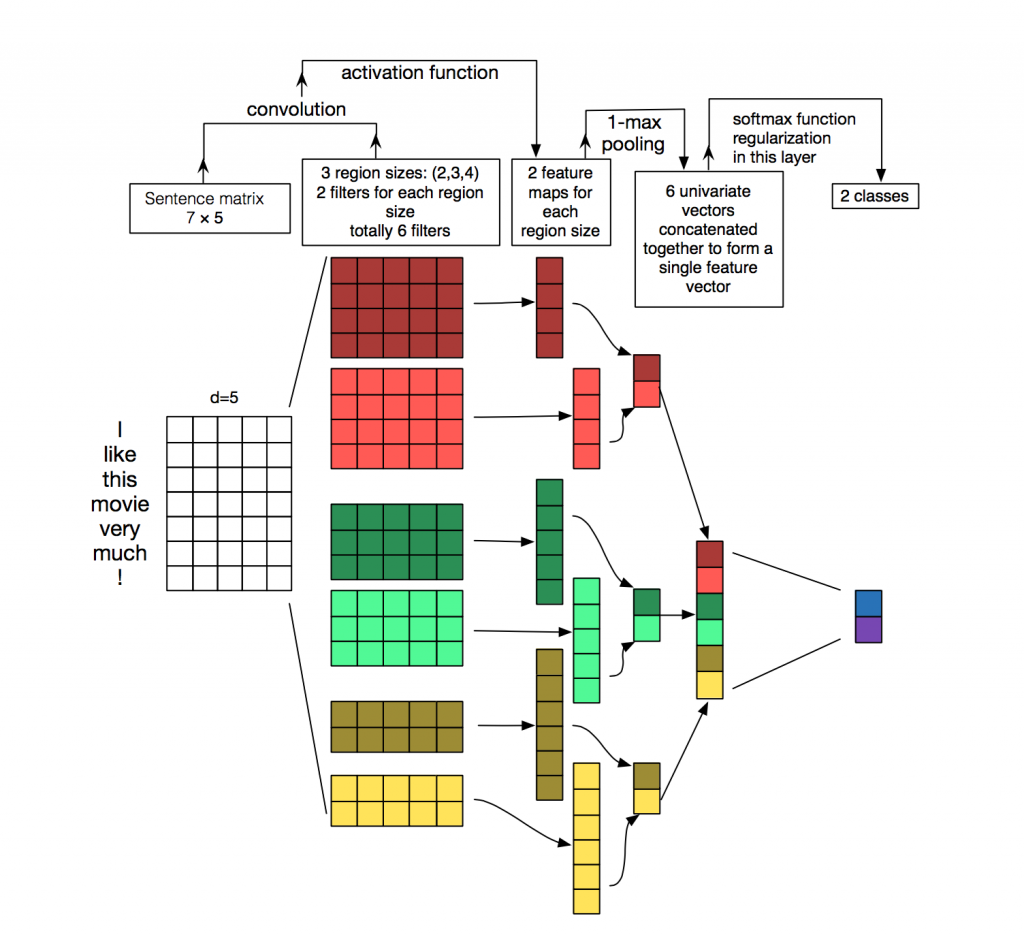# TextRCNN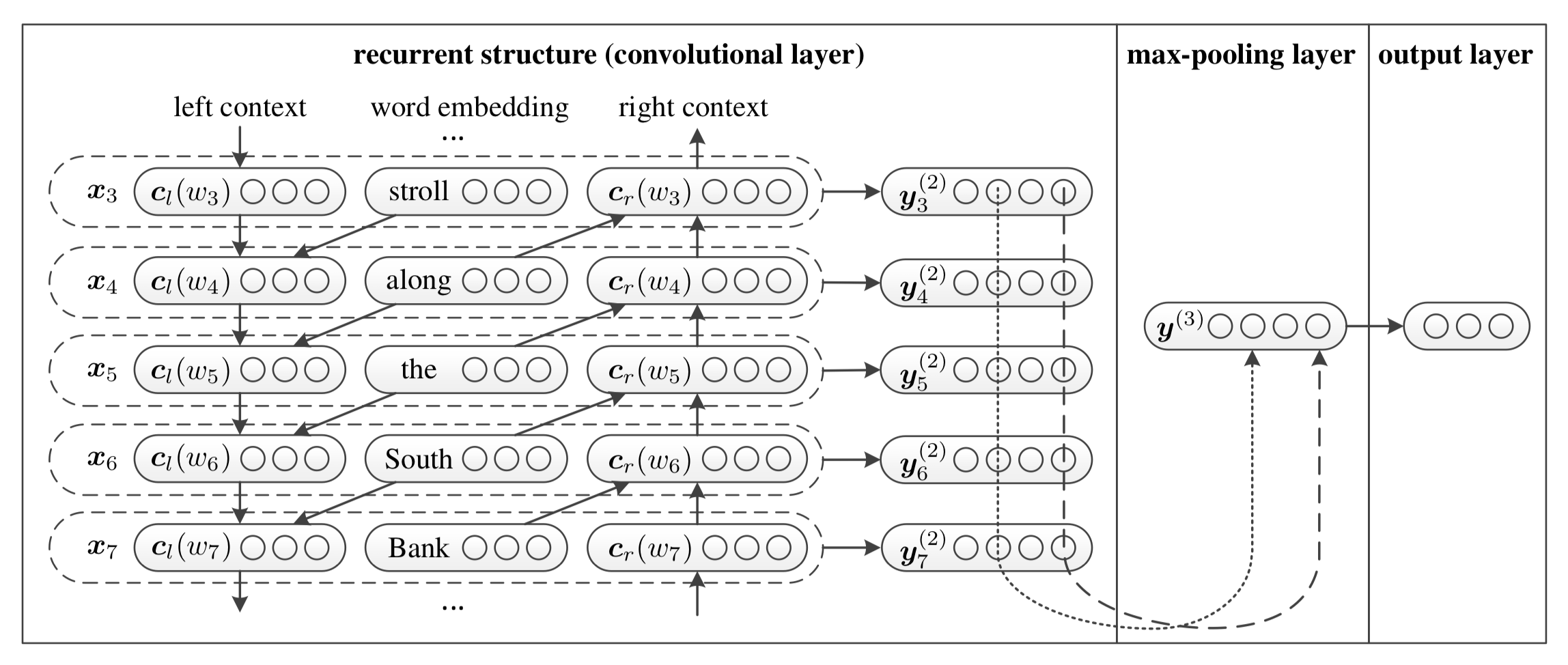• 首先获得词向量表示$$e(w_i)$$
• 其次将词向量通过双向RNN(实践中可以是LSTM或GRU)得到$$c_l(w_i)$$$$c_r(w_i)$$
• $$c_l(w_i)$$, $$e(w_i)$$以及$$c_r(w_i)$$拼接得到新的向量，将其输入到全连接网络对其进行整合，激活函数为tanh
• 再将全连接网络的输出进行MaxPooling
• 最后将其输入一个全连接分类器中实现分类

# FastText

bigram：中华 华人 人民 民共 共和 和国
trigram：中华人 华人民 人民共 民共和 共和国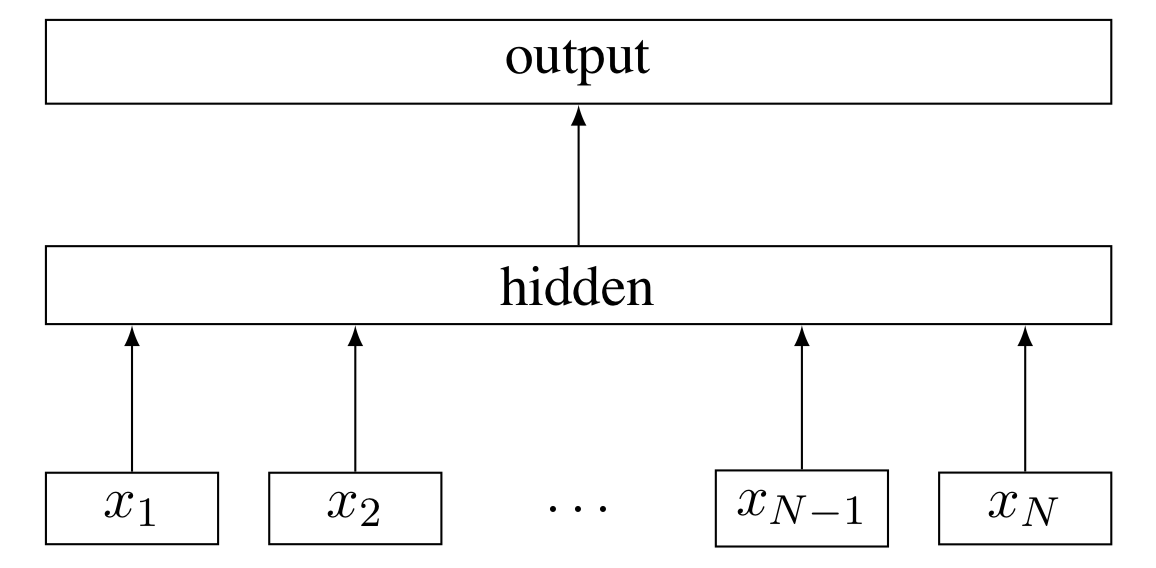# HAN

HAN的全称为Hierarchical Attention Network(分级注意网络)，从字面意思就可以理解其是一个分层架构模型。该模型主要用于文档分类(长文本分类)，其主要结构如下所示：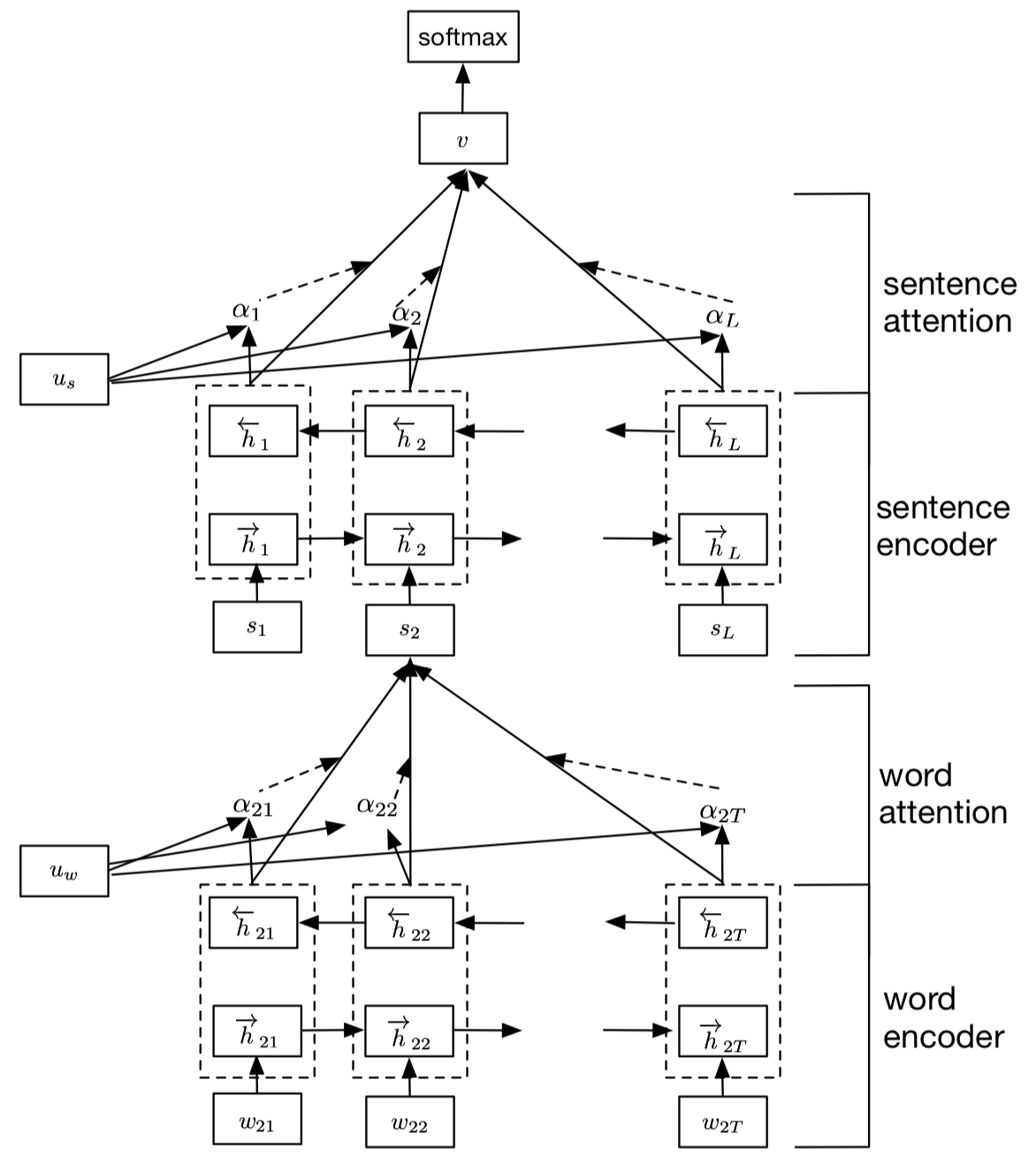HAN主要由两个层次的模型架构：词级别和句级别，每个层次的模型都包括一个编码器和注意力模型两个部分，整个模型的细节如下：

• 如上图所示，假设我们的文档中有$$L$$个句子，对于第二个句子，单词长度为$$T$$
• 首先将单词输入Embedding层获取词向量
• 将获取的词向量输入词编码器，即一个双向GRU，将两个方向的GRU输出拼接在一起得到词级别的隐向量$$h$$
• 将词级别的隐向量通过一个单层感知机(MLP，实际上就是全连接神经网络，激活函数选用tanh)，输出的结果可以看作是更高层次的隐向量表示：
$$u_{it}=tanh(W_wu_{it}+b_w)$$
• 随机初始化一个上下文向量$$u_w$$(随着训练不断优化)，将该上下文向量$$u_w$$与高层次的隐向量表示$$u_{it}$$输入softmax，得到每个词与上下文向量的相似度表示：
$$\alpha_{it}=\frac{exp(u_{it}^Tu_w)}{\sum_texp(u_{it}^Tu_w)}$$
• 将上述相似度作为权重，对$$h_{it}$$加权求和得到句子级别的向量表示：
$$s_i=\sum_t\alpha_{it}h_{it}$$
• 对于句子级别的向量，我们用相类似的方法，将其通过编码层，注意力层，最后将文档中所有句子的隐向量表示加权求和，得到整个文档的文档向量$$v$$，将该向量通过一个全连接分类器进行分类。

# Highway Networks

$y = H(x, W_H)$

$y = H(x, W_H) \cdot T(x, W_T) + x \cdot (1-T(x, W_T))$

$y = \begin{cases} x, & if\ T(x, W_T) = 0 \\ H(x, W_H), & if \ T(x, W_T) = 1 \end{cases}$

posted @ 2019-10-18 16:36  西多士NLP  阅读(11961)  评论(0编辑  收藏  举报